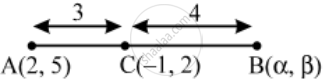# If the point C (–1, 2) divides internally the line segment joining A (2, 5) and B in ratio 3 : 4, find the coordinates of B - Mathematics

Sum

If the point C (–1, 2) divides internally the line segment joining A (2, 5) and B in ratio 3 : 4, find the coordinates of B

#### Solution

Let the coordinates of B be (α, β). It is given that AC : BC = 3 : 4. So, the coordinates of C are( \frac{3\alpha +4\times 2}{3+4},\ \frac{3\beta +4\times 5}{3+4})=( \frac{3\alpha +8}{7},\ \frac{3\beta +20}{7})

But, the coordinates of C are (–1, 2)

\therefore \frac{3\alpha +8}{7}=-1\text{ and }\frac{3\beta +20}{7}=2

⇒ α = – 5 and β = – 2

Thus, the coordinates of B are (–5, –2).

Concept: Section Formula
Is there an error in this question or solution?

Share